## Tuesday, March 31, 2009

### 1784

1784 = 23 x 223.

1784 is a deficient number because the sum of its proper divisors (1 + 2 + 4 + 8 + 223 + 446 + 892 = 1576) is less than the number itself.

1784 is a year in which the month of February has five Sundays.

1784 is a number n such that n2 + 1 and n2 + 3 are both prime: 3,182,657 and 3,182,659.

1784 is a composite number such that exactly eight distinct permutations of its digits give primes: 1487, 1847, 4817, 4871, 7481, 7841, 8147, and 8741.The U.S. Congress ratified the Treaty of Paris on Jan. 14,
1784, officially ending the Revolutionary War.

Source:
The Positive Integers

## Monday, March 30, 2009

### 456

456 = 23 x 3 x 19.

456 is the sum of twin primes: 227 + 229 = 456.

456 is the sum of four consecutive primes: 107 + 109 + 113 + 127 = 456.

456 is the number of tournaments with seven vertices.The German submarine U-456 badly damaged the British cruiser HMS Edinburgh with two torpedo hits on April 30, 1942.

## Friday, March 27, 2009

### 261

261 = 32 x 29.

261 has a representation as a sum of two squares: 261 = 62 + 152.

261 is the only three-digit number n for which 2n - n is a prime number.

Inserting the digit 1 between adjacent digits of 261 gives a prime: 21611.In the novel
Contact by Carl Sagan, Dr. Arroway asks Mr. Kitz, "You wanted to keep the first 261 prime numbers secret?"

Source:
Prime Curios!

## Thursday, March 26, 2009

### 39

39 = 3 x 13.

39 is the sum of the numbers 4 through 9: 4 + 5 + 6 + 7 + 8 + 9 = 39.

39 is the sum of the sixth, seventh, and eighth odd numbers: 11 + 13 + 15 = 39.

39 is the smallest number whose sum of digits is larger than the sum of digits of its square (392 = 1521): 3 + 9 = 12; 1 + 5 + 2 + 1 = 9.

39 is the smallest number with six representations as a sum of three distinct primes: 39 = 3 + 5 + 31 = 3 + 7 + 29 = 3 + 13 + 23 = 3 + 17 + 19 = 5 + 11 + 23 = 7 + 13 + 19.

39 is a number that cannot be written as a sum of three squares.

39 is 33 in base 12.The Thirty-Nine Steps is a spy story by John Buchan.

## Wednesday, March 25, 2009

### 178

178 = 2 x 89. Note that 2 and 89 are the smallest and the largest Mersenne prime exponents under 100.

178 has a cube with the same digits as another cube: 1783 = 5,639,752 and 1963 = 7,529,536.

Each internal angle of a regular 180-sided polygon is
178 degrees.

178 has a representation as a sum of two squares: 178 = 32 + 132.The
Heinkel He 178 was the world's first aircraft to fly under turbojet power, and the first practical jet plane.

Source:
Numeropedia

## Tuesday, March 24, 2009

### 563

563 is a prime number.

563 is the largest known Wilson prime; p is a Wilson prime if p2 divides (p - 1)! + 1. For example, 5 is a Wilson prime because 25 divides 4! + 1 = 25.

Inserting a 9 between adjacent digits of 563 produces a prime: 59693.

563 is a prime such that the number plus its digit sum produces another prime: 563 + 14 = 577, a prime.Reggie Jackson hit 563 home runs in his professional baseball career. He led his teams to five world championships and eleven division titles.

Source: Prime Curios!

## Monday, March 23, 2009

### 328

328 = 23 x 41.

328 is the sum of the first 15 primes.

328 reversed (823) is a prime number.

328 concatenated with its successor is a square: 328329 = 5732.

328 is 101001000 in base 2 (binary).

328 has a representation as a sum of two squares: 328 = 22 + 142.The Ferrari 328 was a sports car manufactured in the late 1980s.

## Friday, March 20, 2009

### 31

31 is a prime number. It is also a Mersenne number (of the form 2p - 1, where p = 5).

31 is the sum of the first two primes raised to themselves: 31 = 22 + 33.

31 is the smallest number with exactly two representations as a sum of four squares: 31 = 12 + 12 + 22 + 52 = 22 + 32 + 32 + 32.

There are only
31 numbers that cannot be expressed as the sum of distinct squares.

29 and 31 form a twin prime pair.

31 is 11111 in base 2 (binary).

31 = 50 + 51 + 52, which means it is 111 in base 5.

31 is a number that cannot be written as a sum of three squares.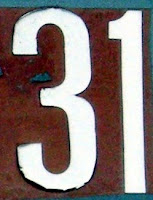31 is the minimum number of moves required to solve a Tower of Hanoi puzzle containing five discs. The Tower of Hanoi puzzle consists of three rods and a given number of discs that can slide onto any rod. The discs start as an ordered stack on one rod, with the largest at the bottom and the smallest on top. The objective is to move all the discs from one rod to another rod, with the discs again stacked in the correct order, subject to the following rules: only one disc may be moved at a time; each move consists of taking the upper disk from one of the rods and sliding it onto another rod, on top of the other disks that may already be present on that rod; and no disc may be placed atop a smaller disc.Source:
Number Gossip; Cut the Knot

## Thursday, March 19, 2009

### 1384

1384 = 23 x 174.

1384 has a representation as a sum of two squares: 1384 = 222 + 302.

1384 has the same digits as the 1384th prime number.

1384 is a number such that the set of base 5 digits of 1384 (21014) equals the set of base 6 digits (10224) of 1384.An
annular solar eclipse occurred on Aug. 17, 1384.

## Wednesday, March 18, 2009

### 755

755 = 5 x 151.

755 is the number of trees on 14 vertices with diameter 6.

755 is the sum of three distinct positive cubes: 755 = 83 + 63 + 33.

755 is the sum of the fifth powers of primes.In 1976, Major League Baseball player Hank Aaron ended his career with a record 755 home runs.

Source: Wikipedia

## Tuesday, March 17, 2009

### 42

42 = 2 x 3 x 7.

42 is a Catalan number.

42 lies between a twin prime pair: 41, 43.

42 is the number of spots on a pair of dice.

42 is 101010 in base 2 (binary).

42 has a unique representation as a sum of three squares: 42 = 12 + 42 + 52.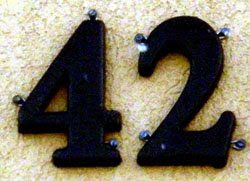Douglas Adams used 42 as "the answer to the ultimate question of life, the universe, and everything" in his Hitchhiker's Guide to the Galaxy series.

Source: Number Gossip

## Monday, March 16, 2009

### 329

329 = 7 x 47.

2
329 is the smallest 100-digit power of 2.

329 is the number of forests with 10 vertices.

329 is the sum of three consecutive primes: 107 + 109 + 113 = 329.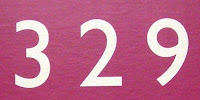329 Svea is a large asteroid discovered in 1892.

Source: Number Gossip

## Friday, March 13, 2009

### 97

97 is the largest two-digit prime number.

97 is the smallest prime that is the sum of a prime number of consecutive primes as well as the sum of a composite number of consecutive composite numbers: 97 = 29 + 31 + 37 = 22 + 24 + 25 + 26.

The first four pairs of digits following the decimal point of 1/97 are powers of three: 1/97 = 0.01030927. . . .

97 has a representation as a sum of two squares: 97 = 42 + 92.The Gregorian calendar has 97 leap days every 400 years.

Source: Prime Curios!

## Thursday, March 12, 2009

### 731

731 = 17 x 43.

731 is the sum of three consecutive primes: 239 + 241 + 251 = 731.

731 is a composite number, being the product of the primes 17 and 43, but 7331, 73331, 733331, and 7333331 are all prime; 731 is the only three-digit composite number with this property.

731 is the largest composite number such that deleting any digit(s) will never yield another composite number. In other words, deletions yield only primes or 1.Unit 731 was a covert biological and chemical warfare research and development unit of the Imperial Japanese Army.

Source: Prime Curios!

## Wednesday, March 11, 2009

### 2701

2701 = 37 x 73.

2701 is a semiprime (a product of two primes) whose prime factors, 37 and 73, are reversals of each other.

2701 is the 73rd triangular number.

2701 is the smallest number n that divides the average of the nth prime and the primes on either side of it.

2701 has two representations as a sum of two squares: 2701 = 102 + 512 = 262 + 452.

2701 is the hypotenuse of two primitive Pythagorean triples: 27 012 = 10202 + 25012 = 13492 + 23402.Source:
Prime Curios!

## Tuesday, March 10, 2009

### 264

264 = 23 x 3 x 11.

264 is the largest known number whose square is undulating: 2642 = 69696.

264 = 25 + 63 + 42. Note that 2 is raised to the third prime, 6 to the second prime, and 4 to the first prime.

264 is a number n such that n2 + 1 is a prime: 2642 + 1 = 69697.

264 is a number that can be expressed as the difference of the squares of primes in just one distinct way: 264 = 172 - 52.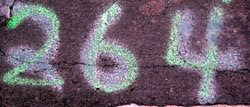The subatomic particle known as the neutral pion has a mass about
264 times that of the electron.

Source:
Prime Curios!

## Monday, March 9, 2009

### 22

22 = 2 x 11.

22 is a pentagonal number.22 is the smallest number that can be expressed as the sum of two primes in three ways: 22 = 3 + 19 = 5 + 17 = 11 + 11.

22 is the number of integer partitions of 8.

22 is the smallest multidigit number such that the sum of its digits equals the product of its digits: 2 + 2 = 2 x 2.

22 has a unique representation as a sum of three squares: 22 = 22 + 32 + 32.The Hebrew alphabet has
22 letters.

Source:
Number Gossip

## Friday, March 6, 2009

### 65

65 = 5 x 13.

65 is the smallest number that becomes square if its reverse is either added to or subtracted from it: 65 + 56 = 121 = 112; 65 - 56 = 9 = 32.

65 is the smallest hypotenuse of two different primitive Pythagorean triangles (33, 56, 65 and 16, 63, 65).

65 is the second number to be the sum of two squares in two ways: 65 = 82 + 12 = 72 + 42. It is the first such number to be the sum of two cubes: 65 = 43 + 13.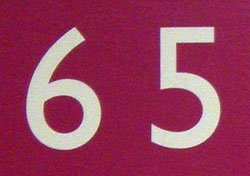65 is the magic constant of the 5 by 5 magic square.

Source: Number Gossip

## Thursday, March 5, 2009

### 336

336 = 24 x 3 x 7.

336 = 6 x 7 x 8.

The product 319530 has the same digits as its factors
336 x 951.

In base 3,
336 is 110110.

336 has a unique representation as a sum of three squares: 336 = 42 + 82 + 162.336 is the number of different moves a knight can make on a chessboard.

Source:
Number Gossip

## Wednesday, March 4, 2009

### 2641

2641 = 19 x 139.

2641 is a number n such that n, n + 1, and n + 2 are products of two primes: n + 1 = 2642 = 2 x 1321 and n + 2 = 2643 = 3 x 881.

2641 is a pseudosquare modulo 11. It is the first pseudosquare that is not prime.

2641 is a centered pentagonal number.A Room with a View by E.M Forester is Project Gutenberg's book 2641.

Source:
Prime Curios!

## Tuesday, March 3, 2009

### 480

480 = 25 x 3 x 5,

480 is the smallest number that can be written as the difference of two squares in eight ways.

480 is the sum of the twin primes 239 and 241 and the sum of four consecutive primes: 109 + 113 + 127 + 131 = 480.

480 has a unique representation as a sum of three squares: 480 = 42 + 82 + 202.480 Hansa is a minor planet orbiting the Sun and the namesake for the Hansa family of high-inclination asteroids.

## Monday, March 2, 2009

### 27

27 = 33, a perfect cube.

27 is the largest number that is the sum of the digits of its cube: 273 = 19683; 1 + 9 + 6 + 8 + 3 = 27.

27 is the only number that is three times the sum of its digits.

27 is 11011 in base 2 (binary) and 33 in base 8.

27 is the smallest number with two representations as a sum of three squares: 27 = 12 + 12 + 52 = 32 + 32 + 32.

27 is the number of integer partitions of 15 into distinct parts.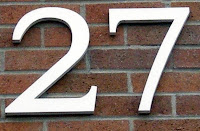The chemical element
cobalt has atomic number 27.

Source:
Number Gossip